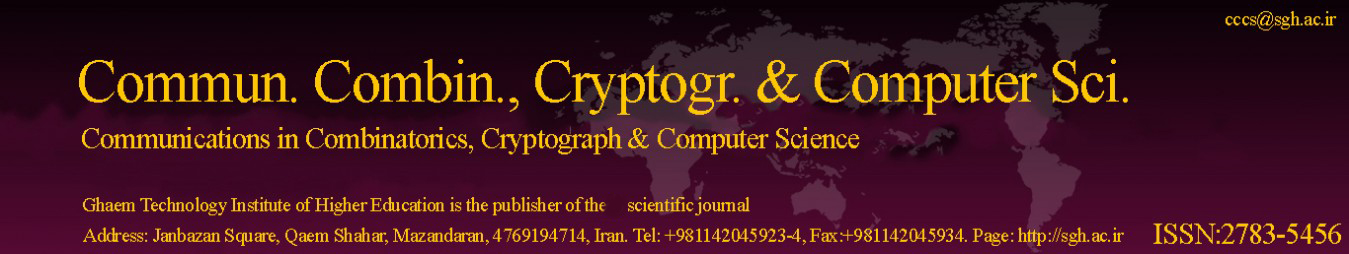Original Research Article

Article volume = 2022 and issue = 1

Pages: 71–78

Article publication Date: November 21, 2022

# Anti Fuzzy Congruence on Product Lattices

#### Rasul Rasuli

Department of Mathematics, Payame Noor University(PNU), P. O. Box 19395-4697, Tehran, Iran.

##### Abstract:

In this work, the concept of anti fuzzy congruence on lattice $L$ is introduced. Also if $K$ be another lattice, then the concept of anti fuzzy congruence on the product $L \times K$ is discussed. Finally it is proved for every anti fuzzy congruence relation $\mu$ on $L \times K,$ the anti fuzzy congruences $\mu_{L}$ and $\mu_{K}$ can be defined on $L$ and $K$ respectively such that $\mu=\mu_{L} \times \mu_{K}$.

##### Keywords:

Fuzzy set theory, Lattices and related structures, Congruence relations, Direct product, Isomorphisms.

##### References:
•  R. Belohlavek, Fuzzy Relational Systems, Kluwer Academic Publishers, Dordrecht, 2002. 1
•  M. Ciric, J. Ignjatovic and S. Bogdanovic, Uniform fuzzy relations and fuzzy functions, Fuzzy Sets and Systems, 160 (2009), 1054-1081. 1
•  P. Das, Lattice of fuzzy congruences in inverse semigroups, Fuzzy Sets and Systems, 91(1997), 399-408. 1
•  A. Di Nola, S. Sessa, W. Pedrycz and E. Sanchez, Fuzzy Relation Equations and Their Applications to Knowledge Engineering, in: Theory and Decision Libr., Ser. D, Kluwer Acad. Publ., Dordrecht, 1989. 1
•  J. Goguen, L-fuzzy sets, Mathematics Analisys and Applications, 18(1967), 145-174. 1
•  G. Gratzer, Lattice Theory: Foundation, University of Manitoba, Canada, 2011. 2.1, 2.2, 2.3, 2.4
•  P. Hajek, Basic fuzzy logic and BL-algebras, Soft Computing, 2(1998), 124-128. 1
•  J. Ignjatovic, M. Ciric and S. Bogdanovic, On the greatest solutions to weakly linear systems of fuzzy relation inequalities and equations, Fuzzy Sets and Systems, 161 (2010), 3081-3113. 1
•  G. J. Klir and B. Yuan, Fuzzy Sets and Fuzzy Logic, Prentice Hal, Upper Saddle River, NJ, 1995. 1
•  D. S. Malik and J. N. Mordeson, Fuzzy Commutative Algebra, World Science publishing Co.Pte.Ltd.,(1995). 2.5
•  R. Rasuli, Fuzzy Sub-vector Spaces and Sub-bivector Spaces under t-Norms, General Letters in Mathematics, 5(2018), 47-57. 4
•  R. Rasuli, Conorms over conjugates and generalized characterestics of anti Q-fuzzy subgroups, 3rd national Conference on Management and Fuzzy Systems, University of Eyvanekey, Eyvanekey, Iran, March 2021. 4
•  R. Rasuli, Fuzzy Relations on Modules under T-norms, The Fourth International Conference on Soft Computing( CSC), University of Guilan, December 29-30, 2021. 4
•  R. Rasuli, M. M. Moatamedi nezhad and H. Naraghi, Anti Fuzzy SU-subalgebra under conorms, The Third National Congress on Mathematics and Statistics Conbad Kavous University, 2021. 4
•  R. Rasuli, M. M. Moatamedi nezhad and H. Naraghi, S-norms over anti fuzzy implicative ideals, anti fuzzy positive imoplicative ideals in BCK-Algebras, The Third National Congress on Mathematics and Statistics Conbad Kavous University, 2021. 4
•  R. Rasuli, Fuzzy congruence on product lattices under T-norms, Journal of Information and Optimization Sciences, 42(2)(2021), 333-343. 4
•  R. Rasuli, Intuitionistic fuzzy congruences on product lattices under norms, Journal of Interdisciplinary Mathematics, 24(5)(2021), 1281-1304. 4
•  R. Rasuli, Conorms over level subsets and translations of anti Q-fuzzy Subgroups, International Journal of Mathematics and Computation, 32(2)(2021), 55-67. 4
•  R. Rasuli, Norms on intuitionistic fuzzy muligroups, Yugoslav Journal of Operations Research, 31(3)(2021), 339-362. 4
•  R. Rasuli, Norms on intuitionistic fuzzy congruence relations on rings, Notes on Intuitionistic Fuzzy Sets, 27(3)(2021), 51-68. 4
•  R. Rasuli, Norms on intuitionistic fuzzy SU-subalgebras, Scientia Magna, 16(1)(2021), 84-96. 4
•  R. Rasuli, Bifuzzy d-algebras under norms, Mathematical Analysis and its Contemporary Applications, 3(4)(2021), 63-83. 4
•  R. Rasuli, Strongest relation, cosets and middle cosets of AQFSC(G), Eng. Appl. Sci. Lett. (EASL), 4(3)(2021), 1-7. 4
•  R. Rasuli, S-norms on anti Q-fuzzy subgroups, Open J. Discret. Appl. Math., 4(3)(2021), 1-9. 4
•  R. Rasuli, Intuitionistic fuzzy congruences on product lattices, J. of Ramannujan Society of Mathematics and Mathematical Sciences, 9(1)(2021), 125-144. 4
•  R. Rasuli, t-norms over fuzzy ideals (implicative, positive implicative) of BCK-algebras, Mathematical Analysis and its Contemporary Applications, 4(2)(2022), 17-34. 4
•  R. Rasuli, T-fuzzy subbigroups and normal T-fuzzy subbigroups of bigroups, J. of Ramannujan Society of Mathematics and Mathematical Sciences, 9(2)(2022), 165-184. 4
•  R. Rasuli, M. A. Hashemi and B. Taherkhani, S-norms and Anti fuzzy ideals of BCI-algebras, 10th National Mathematics Conference of the Payame Noor University, Shiraz, May, 2022. 4
•  R. Rasuli, B. Taherkhani and H. Naraghi, T-fuzzy SU-subalgebras, 10th National Mathematics Conference of the Payame Noor University, Shiraz, May, 2022. 4
•  R. Rasuli, A study of T-fuzzy multigroups and direct preoduct of them, 1th National Conference on Applied Reserches in Basic Sciences( Mathematics, Chemistry and Physics) held by University of Ayatolla Boroujerdi, Iran, during May 26-27, 2022. 4
•  R. Rasuli, S - (M,N)-fuzzy subgroups, 1th National Conference on Applied Reserches in Basic Sciences( Mathematics, Chemistry and Physics) held by University of Ayatolla Boroujerdi, Iran, during May 26-27, 2022. 4
•  R. Rasuli, Intuitionistic fuzzy BCI-algebras (implicative ideals, closed implicative ideals, commutative ideals) under norms, Mathematical Analysis and its Contemporary Applications, 4(3)(2022), 17-34. 4
•  E. Turunen, Mathematics Behind Fuzzy Logic, Physica-Verlag, Heidelberg, 1999. 1
•  Z. D. Wang and Y. D. Yu, Pseudo t-norms and implication operators on a complete brouwerian lattice, Fuzzy Sets and Systems, 132(2002), 113-124. 1
•  T. Yijia, Fuzzy congruences on a regular semigroup, Fuzzy Sets and Systems, 117(2001), 447-453. 1
•  L. A. Zadeh, Fuzzy probabilities, Information Processing and Management, 20(1984), 363-372. 1